Latest Teaching jobs   »   Linear Equations- Mathematics Study Notes For...

# Linear Equations- Mathematics Study Notes For CTET Exam: Download Free PDFMathematics is an equally important section for CTET, MPTET, HTET Exams and has even more abundant importance in some other exams conducted by central or state govt. Generally, there are questions asked related to basic concepts, Facts and Formulae of the Linear Equations.

Practice Maths with CTET Latest Study Plan For 60 Days

To let you make the most of Mathematics section, we are providing important facts related to the Linear Equations. At least 1-2 questions are asked from this topic in most of the teaching exams. We wish you all the best of luck to come over the fear of the Mathematics section.

How to Overcome Exam Fever, Especially When You Fear Maths

## Linear Equations

Linear Equations in Two variables: An equation of the form ax+ by + c = 0 where a, b, c ∈ R (real numbers) and a ≠ 0, b ≠ 0 and x, y are variables is called linear equation in two variables.

Examples: Each of the following equations is a linear equation:
(i) 4x + 7y – 13
(ii) 2x – 5y = 36
(iii) x – y = 2

Ex. One number is thrice the other number. When the large number is Subtracted from 49, the result is two more than the smaller number subtracted from 43. Find the numbers

Sol. Let a number = x
then second number = 3x
49 – 3x = (43 – x) + 2
49 – 3x = 45 – x
4 = 2x
x = 2, Number ⟹ 2, 6

• The condition a ≠ 0, b ≠ 0, is often denoted by a² + b² ≠ 0
• The graph of a linear equation ax + by + c = 0, is a straight line

Mathematics Study Notes For All Teaching Exams

Solution of linear equation: Any pair of values of x and y which satisfy the equation ax + by + c = 0, is called its solution.

Ex. show that x = 2 and y = 1 is a solution of 2x + 5y = 9
Sol: Substituting x = 2 and y = 1 in the given equation, we get RHS
= 2 × 2 + 5 × 1 = 9
∴ x =2, y = 1 is a solution of 2x + 5y = 9
Ex. If 9x – 11 = -2x + 52, then find the value of x.
Sol. 9x -11 = -2x + 52
11x = 63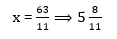Mathematics Quiz For CTET Exam : Attempt Daily Quizzes

## System of Linear Equations:

Consistent System: A system consisting of two simultaneous, linear equations is said to be consistent, if it has at least one solution.

Inconsistent System: A system consisting simultaneous linear equations is said to be inconsistent, if It has no solution at all.

Ex. Consider the system of equations: x + y = 9 & 3x + 3y = 5. Clearly, there are no values of x and y which may simultaneously satisfy the given equations. So, the system given above is inconsistent.

Conditions for Solvability: The system of equations a₁x + b₁y + c₁ = 0, a₂x + b₂y + c₂ = 0 has: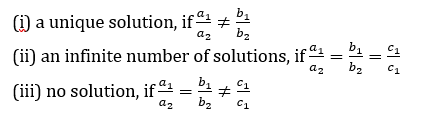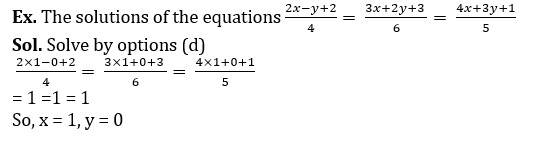Homogenous System of Equations: The system of equations a₁e + b₁y = 0; a₂r + b₂y = 0 has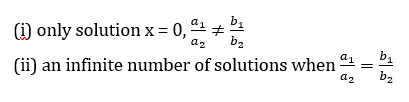Practice More Mathematics  Quiz Here
The graphs of a₁x + b₁y + c₁= 0, a₂x b₂y + c₂ = 0 will be:
(i) parallel, if the system has no Solution;
(ii) coincident, if the system has infinite number of solutions;
(iii) Intersecting, if the system has a unique solution.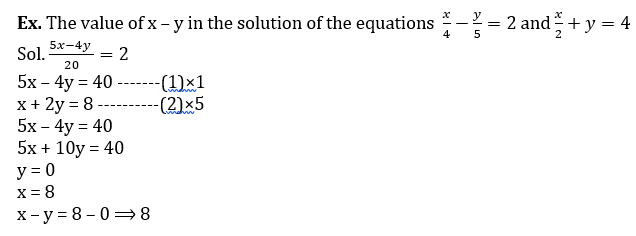•Learning Curve - What is Learning Curve?...
•रस - परिभाषा, भे�...
•पठन कौशल- परिभा�...
•अलंकार - परिभाष�...
•Indian States and Capitals 2022: 28 Stat...
•New Education Policy(NEP) PDF - नई �...